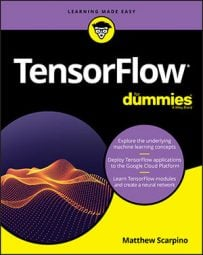##### TensorFlow For DummiesMachine learning applications store a great deal of data in vectors (one-dimensional tensors) and matrices (two-dimensional tensors). To process this data, TensorFlow provides many functions that operate on vectors and matrices. The following table lists these functions and provides a description of each.

Vector and Matrix Operations

 Function Description `tensordot(a, b, axes, name=None)` Returns the sum of products for the elements in the given axes `cross(a, b, name=None)` Returns the element-wise cross product `diag(diagonal, name=None)` Returns a matrix with the given diagonal values, other values set to zero `trace(x, name=None)` Returns the sum of the diagonal elements `transpose(x, perm=None,``name='transpose')` Switches rows and columns `eye(num_rows, num_columns=None,``batch_shape=None,``dtype=tf.float32,``name=None)` Creates an identity matrix with the given shape and data type `matmul(a, b,``transpose_a=False,``transpose_b=False,``adjoint_a=False,``adjoint_b=False,``a_is_sparse=False,``b_is_sparse=False,``name=None)` Returns the product of the two input matrices `norm(tensor,``ord='euclidean',``axis=None,``keep_dims=False,``name=None)` Returns the norm of the given axis of the input tensor with the specified order `matrix_solve(A, b,``adjoint=None,``name=None)` Returns the tensor x, such that Ax = b, where A is a matrix, and b is a vector `qr(input, full_matrices=None,``name=None)` Returns the eigenvectors and eigenvalues of the given matrix or matrices `svd(tensor,``full_matrices=False,``compute_uv=True,``name=None)` Factors the matrix into a unitary matrix, a diagonal matrix, and the conjugate transpose of the unitary matrix `einsum(equation, *inputs)` Executes a custom mathematical operation
Of these functions, the two most common are `tensordot `and `matmul`. `tensordot `returns the dot product of one or more axes of two input tensors. That is, `tensordot `multiplies the corresponding elements of both tensors' dimensions and returns the sum of the products.

The `axes `parameter tells `tensordot `which dimensions to process. If you set this parameter to a scalar, N, the function will access the last N axes of the first tensor and the first N axes of the second tensor. If you set `axes `equal to a list or tensor, the first row identifies axes of the first tensor, and the second row identifies axes of the second tensor.

I frequently call `tensordot `to compute the dot product of two one-dimensional tensors. The following code shows what this looks like:

`t1 = tf.constant([4., 3., 2.])t2 = tf.constant([3., 2., 1.])dot = tf.tensordot(t1, t2, 1)<strong># 4*3 + 3*2 + 2*1 = 20</strong>`
`matmul `performs traditional matrix multiplication. That is, it multiplies rows of the first tensor by columns of the second tensor and returns a matrix containing the sums. The following code shows how this can be used:
`t1 = tf.constant([[1.0, 2.0, 3.0], [4.0, 5.0, 6.0]])t2 = tf.constant([[1.0, 2.0], [3.0, 4.0], [5.0, 6.0]])dot = tf.matmul(t1, t2)<strong># [[ 22. 28.], [ 49. 64.]]</strong>`
My favorite function in the table is `einsum`, which makes it possible to create and execute custom mathematical operations. The first parameter is a string that identifies the operation using a special format called the Einstein summation convention. This convention has a number of characteristics, including the following:
• The operation is assumed to have one or two inputs. If you provide two inputs, you must separate them with a comma.
• Dimensions of input and output matrices are represented by subscripts (usually `i`, `j`, and `k`). Input subscripts must be separated from output subscripts with the → symbol.
• If an input's subscript is repeated and no output subscripts are given, the operation performs addition. Therefore, `einsum('i,i', t1, t2)` computes the dot product of tensors `t1` and `t2`.
• If an input's subscript is repeated and output subscripts are given, the operation performs multiplication. Therefore, `einsum('i,i->i', t1, t2) `computes the element-wise product of tensors `t1` and `t2`.
The following code calls `einsum `to transpose a matrix and multiply two matrices together:
`m1 = tf.constant([[1, 2], [3, 4]])m2 = tf.constant([[5, 6], [7, 8]])e1 = tf.einsum'ij-<ji', m1)     # [[1, 3], [2, 4]]e2 = tf.einsum('ij,jk-<ik', m1, m2) # [[19, 22], [43, 50]]`
For a more complete discussion of the Einstein summation convention, try Samuel Prime's presentation.